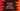# How to add elements to the start of JavaScript arrays by using unshift## How to add elements to the start of JavaScript arrays by using unshift:

The unshift method can be used to add one or more elements to the start or beginning of an array in JavaScript. It adds the elements and modifies the original array.

In this post, we will learn how unshift works with examples.

### Definition of unshift():

The unshift() method is defined as like below:

``arr.unshift(e1, e2, e3....)``

Where,

• arr is the array.
• e1, e2.. etc. are elements to add to the front of the array.

#### Return value of unshift():

It returns the final length of the array after all elements are added.

### Example of unshift():

Let’s learn how unshift works with an example:

``````let givenArray = [1, 2, 3];

console.log('givenArray: ', givenArray);

givenArray.unshift(0);
console.log('unshift(0): ', givenArray);

givenArray.unshift(-1);
console.log('unshift(-1): ', givenArray);

givenArray.unshift(-2);
console.log('unshift(-2): ', givenArray);``````

Here,

• givenArray is the given array.
• It uses unshift three times and prints the result.

If you run this, it will print the below output:

``````givenArray:  [ 1, 2, 3 ]
unshift(0):  [ 0, 1, 2, 3 ]
unshift(-1):  [ -1, 0, 1, 2, 3 ]
unshift(-2):  [ -2, -1, 0, 1, 2, 3 ]``````

You can see here that on each unshift, it adds the value to the left.

### Example of unshift() return value:

As explained above, unshift returns the length of the new array. For example,

``````let givenArray = [1, 2, 3];

console.log('givenArray: ', givenArray);

console.log(givenArray.unshift(0));
console.log(givenArray.unshift(-1));
console.log(givenArray.unshift(-2));``````

It will print:

``````givenArray:  [ 1, 2, 3 ]
4
5
6``````

You can see that each time unshift is called, it prints the new array length. The starting array length is 3, after first unshift, it is 4 etc. So, it always returns the final array length.

### Example of unshift with objects:

Let’s take a look on unshift with objects:

``````let givenArray = [{ first: 1 }, { second: 2 }, { third: 3 }];

console.log("givenArray: ", givenArray);

givenArray.unshift({ fourth: 4 });

console.log("givenArray after unshift: ",givenArray);``````

It will print the below output:

``````givenArray:  [ { first: 1 }, { second: 2 }, { third: 3 } ]
givenArray after unshift:  [ { fourth: 4 }, { first: 1 }, { second: 2 }, { third: 3 } ]``````

As you can see here, it works similarly to the previous examples.